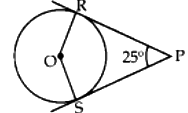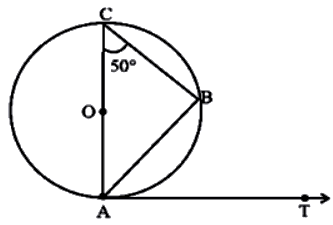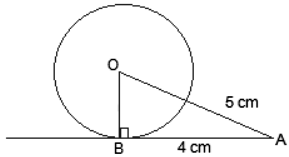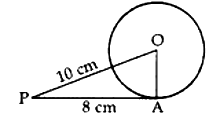Check the underneath Class 10 Maths MCQ Questions of Circles with Answers. These MCQ Questions for Class 10 Maths with Answers were ready for students and it’s most vital for exams. We have given MCQ Questions of Circles with Answers to assist students with understanding the idea well overall. Understudies can tackle Class 10 Maths Circles MCQs with Answers to realize their Knowledge level.

Students can likewise refer to MCQ Questions for Class 10 for better exam preparation and score more numbers in exams.

1. A……..is a collection of all points in a plane which are at a constant distance (radius) from a fixed point (centre).

(a) Circle
(b) Oval
(c) Parallelogram
(d) None of the above

2. A …… to a circle is a line that intersects the circle at only one point.

(a) Tangent
(b) Secant
(c) Segment
(d) None of the above

3. A circle has a number of tangents equal to

(a) 0
(b) 1
(c) 2
(d) Infinite

4. A tangent intersects the circle at:

(a) One point
(b) Two distinct point
(c) At the circle
(d) None of the above

5. A circle can have _____parallel tangents at a single time.

(a) One
(b) Two
(c) Three
(d) Four

6. If the angle between two radii of a circle is 110°, then the angle between the tangents at the ends of the radii is:

(a) 90°
(b) 50°
(c) 70°
(c) 40°

7. The length of the tangent from an external point A on a circle with centre O is

(a) always greater than OA
(b) equal to OA
(c) always less than OA
(d) Cannot be estimated

8. Tangents from an external point to a circle are

(a) equal
(b) not equal
(c) parallel
(d) perpendicular

9. Two circle touch each other externally at C and AB is a common tangent to the circles. Then, ∠ACB =

(a) 60°
(b) 45°
(c) 30°
(d) 90°

10. A line through point of contact and passing through centre of circle is known as

(a) tangent
(b) chord
(c) normal
(d) segment

11. The tangents drawn at the extremities of the diameter of a circle are

(a) perpendicular
(b) parallel
(c) equal
(d) none of these

12. If four sides of a quadrilateral ABCD are tangential to a circle, then

(a) AC + AD = BD + CD
(b) AB + CD = BC + AD
(c) AB + CD = AC + BC
(d) AC + AD = BC + DB

13. AB is a chord of the circle and AOC is its diameter such that angle ACB = 50°. If AT is the tangent to the circle at the point A, then BAT is equal to

(a)65°
(b)60°
(c)50°
(d)40°

14. If a parallelogram circumscribes a circle, then it is a:

(a) Square
(b) Rectangle
(c) Rhombus
(d) None of the above

15. The length of a tangent from a point A at a distance 5 cm from the centre of the circle is 4 cm. The radius of the circle is:

(a) 3cm
(b) 5cm
(c) 7cm
(d) 10cm

16. The distance between two parallel tangents of acircle of radius 4 cm is

(a) 2 cm
(b) 4 cm
(c) 6 cm
(d) 8 cm

17. In the given figure, if ZRPS = 25°, the value of ZROS is(a) 135°
(b) 145°
(c) 165°
(d) 155°

18. Number of tangents drawn at a point of the , circle is/are

(a) one
(b) two
(c) none
(d) infinite

19. The length of a tangent drawn from a point at a distance of 10 cm of circle is 8 cm. The radius of the circle is

(a) 4 cm
(b) 5 cm
(c) 6 cm
(d) 7 cm

20. Two parallel lines touch the circle at points A and B respectively. If area of the circle is 25 n cm$$^2$$, then AB is equal to

(a) 5 cm
(b) 8 cm
(c) 10 cm
(d) 25 cm

Explanation: A circle has infinitely many tangents, touching the circle at infinite points on its circumference.

Explanation: A tangent touches the circle only on its boundary and do not cross through it.

Explanation: A circle can have two parallel tangents at the most.

Explanation: If the angle between two radii of a circle is 110°, then the angle between tangents is 180° − 110° = 70°. (By circles and tangents properties)

7. Answer: (c) always less than OA

Explanation: Since the tangent is perpendicular to the radius of the circle, then the angle between them is 90º. Thus, OA is the hypotenuse for the right triangle OAB, which is right-angled at B. As we know, for any right triangle, the hypotenuse is the longest side. Therefore the length of the tangent from an external point is always less than the OA.

Explanation: According to the question:∠ABC = 90 (Angle in Semicircle)

In ∆ACB

∠A + ∠B + ∠C = 180°

∠A = 180° – (90° + 50°)

∠A = 40°

Or ∠OAB = 40°

Therefore, ∠BAT = 90° – 40° = 50°

Explanation:  According to the given question:AB is the tangent, drawn on the circle from point A.

So, OB ⊥ AB

Given, OA = 5cm and AB = 4 cm

Now, In △ABO,

OA$$^2$$ = AB$$^2$$ + BO$$^2$$ (Using Pythagoras theorem)

⇒ 5$$^2$$ = 4$$^2$$ + BO$$^2$$

⇒ BO$$^2$$ = 25 – 16

⇒ BO$$^2$$ = 9

⇒ BO = 3

Explanation:Here radius, r = 4 cm

Required distance,
AB = OA + OB
= r + r = 2r = 2×4 = 8 cm

Explanation: Since OR ⊥ PR and OS ⊥ PS
∴ ∠ORP = ∠OSP = 90°
In □ ORPS, ∠ROS + ∠ORP + ∠RPS + ∠OSP = 360°
∠ROS + 90° + 25° + 90° = 360°
∠ROS = 360° – 205° = 155°

Explanation: There is only one tangent at a point of the circle.

Explanation:In rt. AOAP, we have
OA$$^2$$ + AP$$^2$$ = OP$$^2$$
⇒ OA$$^2$$ + (8)$$^2$$ = (10)$$^2$$
⇒ OA$$^2$$ + 64 = 100
⇒ OA$$^2$$ = 100 – 64 = 36
∴ OA = √36 = 6 cm

∴ $$\pi R^2$$ = $$25\pi$$